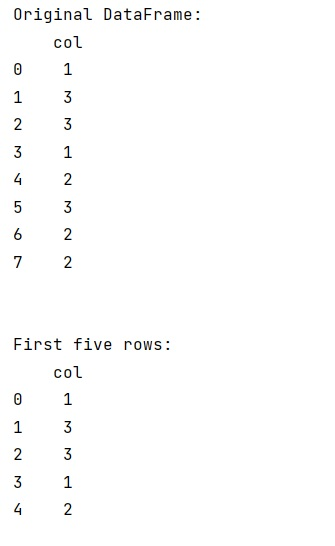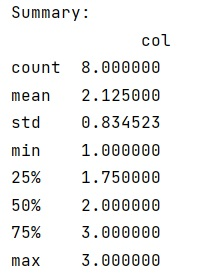# R summary() equivalent in numpy

Learn about the numpy summary which is equivalent to R's summary.
Submitted by Pranit Sharma, on February 16, 2023

NumPy is an abbreviated form of Numerical Python. It is used for different types of scientific operations in python. Numpy is a vast library in python which is used for almost every kind of scientific or mathematical operation. It is itself an array which is a collection of various methods and functions for processing the arrays.

We know that numpy has std, mean, and average, but it does not have a summary() method that sums up everything.

We need to understand if is there any possible way, to sum up everything in numpy or pandas as we can do in R.

The answer to this question is Yes, we can find out the summary of a data set in pandas using the following steps,

Examine the first few rows of data:

## Python code to demonstrate the R summary() equivalent in numpy

Examine the first few rows of data

```# Importing pandas package
import pandas as pd

# Import numpy
import numpy as np

# Creating a dataframe
df = pd.DataFrame({'col':[1,3,3,1,2,3,2,2]})

# Display the DataFrame
print("Original DataFrame:\n",df,"\n\n")

# examine first few rows
```

Output:Calculate summary statistics

```# Calculate summary statistics
print("Summary:\n",df.describe(),"\n")
```

Output: